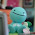## Sunday, January 16, 2011

### Final Percent Post

Percent- means out of 100, it can also be written as a decimal or fraction.

Key points of chapter 4

Representing percents- you can represent percents by using a hundred grid, use one grid if the percent is under a hundred. Or 2 girds if over a hundred, to show a decimal you can shade part of a square in the grid.

Fractions, Percents, and Decimals- decimals can be written as a percent or fraction. Decimal to percent is multiplying by a hundred, and decimal to fraction is writing the decimal over 1 then multiplying the numerator and denominator by 10 for every number after decimal.

Percent of a number-you can find percents of a number by doubling,halving, or dividing by ten.

Combining Percents- percents can be combined by adding to solve problems (5% +7%=12%). To calculate the increase in a number, you can add the combined percent to the original number (12% of 100 = 0.12 x 100=12 100 + 12 = 112). You can also multiply the original number by a single percent greater than 100. (112% of 100 = 1.12 x 100 = 112).

#### 1 comment:

1.I like how you used some bolded/italicized wording but there is no color in it. Also, you should put your video like that was our instructions other than that great job Justin!